` `

# Spezialisierte Gitteranalyse¶

## D8 Abstand zu Strömen¶

### Beschreibung¶

Computes the horizontal distance to stream for each grid cell, moving downslope according to the D8 flow model, until a stream grid cell is encountered.

### Parameter¶

D8 Fließrichtung Raster [raster]
This input is a grid of flow directions that are encoded using the D8 method where all flow from a cells goes to a single neighboring cell in the direction of steepest descent. This grid can be obtained as the output of the “D8 Flow Directions” tool.
Stromrastergitter [raster]
A grid indicating streams. Such a grid can be created by several of the tools in the “Stream Network Analysis” toolset. However, the tools in the “Stream Network Analysis” toolset only create grids with a value of 0 for no stream, or 1 for stream cells. This tool can also accept grids with values greater than 1, which can be used in conjunction with the Threshold parameter to determine the location of streams. This allows Contributing Area grids to be used to define streams as well as the normal Stream Raster grids. This grid expects integer (long integer) values and any non-integer values will be truncated to an integer before being evaluated.
Schwellen [number]

This value acts as threshold on the Stream Raster Grid to determine the location of streams. Cells with a Stream Raster Grid value greater than or equal to the Threshold value are interpreted as streams.

Vorgabe: 50

### Ausgaben¶

Output Distance to Streams [raster]
A grid giving the horizontal distance along the flow path as defined by the D8 Flow Directions Grid to the streams in the Stream Raster Grid.

### Console usage¶

```processing.runalg('taudem:d8distancetostreams', -p, -src, -thresh, -dist)
```

## D-Unendlich Lawinenauslauf¶

### Beschreibung¶

Identifies an avalanche’s affected area and the flow path length to each cell in that affacted area. All cells downslope from each source area cell, up to the point where the slope from the source to the affected area is less than a threshold angle called the Alpha Angle can be in the affected area. This tool uses the D-infinity multiple flow direction method for determining flow direction. This will likely cause very small amounts of flow to be dispersed to some downslope cells that might overstate the affected area, so a threshold proportion can be set to avoid this excess dispersion. The flow path length is the distance from the cell in question to the source cell that has the highest angle.

All points downslope from the source area are potentially in the affected area, but not beyond a point where the slope from the source to the affected area is less than a threshold angle called the Alpha Angle.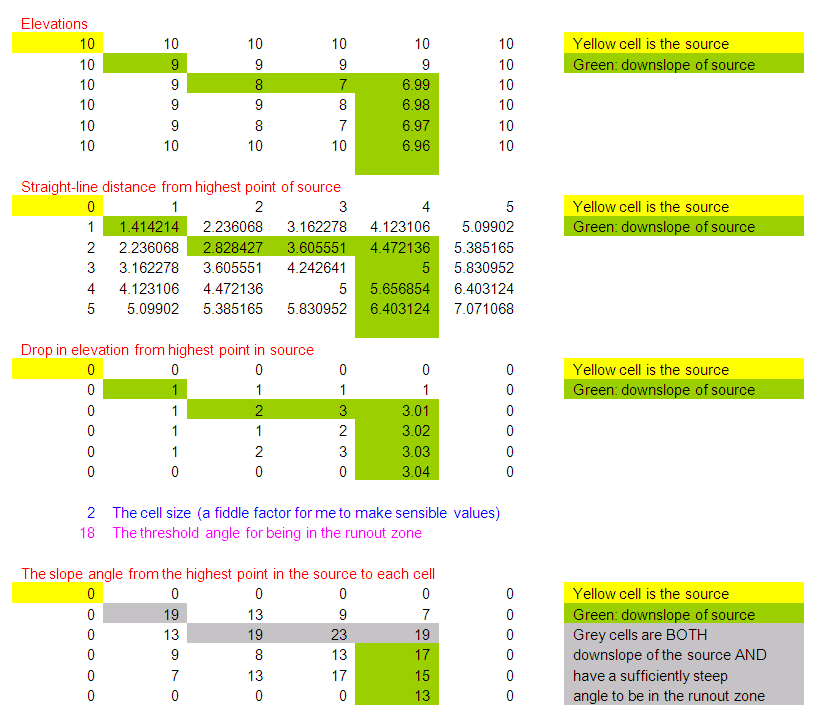Slope is to be measured using the straight line distance from source point to evaluation point.

It makes more physical sense to me for the angle to be measured along the flow path. Nevertheless it is equally easy to code straight line angles as angles along the flow path, so an option that allows switching will be provided. The most practical way to evaluate avalanche runout is to keep track of the source point with the greatest angle to each point. Then the recursive upslope flow algebra approach will look at a grid cell and all its upslope neighbors that flow to it. Information from the upslope neighbors will be used to calculate the angle to the grid cell in question and retain it in the runout zone if the angle exceeds the alpha angle. This procedure makes the assumption that the maximum angle at a grid cell will be from the set of cells that have maximum angles to the inflowing neighbors. This will always be true of angle is calculated along a flow path, but I can conceive of cases where flow paths bend back on themselves where this would not be the case for straight line angles.

The D-infinity multiple flow direction field assigns flow from each grid cell to multiple downslope neighbors using proportions (Pik) that vary between 0 and 1 and sum to 1 for all flows out of a grid cell. It may be desirable to specify a threshold T that this proportion has to exceed before a grid cell is counted as flowing to a downslope grid cell, e.g. Pik > T (=0.2 say) to avoid dispersion to grid cells that get very little flow. T will be specified as a user input. If all upslope grid cells are to be used T may be input as 0.

Avalanche source sites are to be input as a short integer grid (name suffix *ass, e.g. demass) comprised of positive values where avalanches may be triggered and 0 values elsewhere.

The following grids are output:

• rz — A runout zone indicator with value 0 to indicate that this grid cell is not in the runout zone and value > 0 to indicate that this grid cell is in the runout zone. Since there may be information in the angle to the associated source site, this variable will be assigned the angle to the source site (in degrees)
• dm — Along flow distance from the source site that has the highest angle to the point in question

### Parameter¶

D-Infinity Fliessrichtung Raster [raster]
A grid giving flow direction by the D-infinity method. Flow direction is measured in radians, counter clockwise from east. This can be created by the tool “D-Infinity Flow Directions”.
Höhengitter mit gefüllten Löchern [raster]
This input is a grid of elevation values. As a general rule, it is recommended that you use a grid of elevation values that have had the pits removed for this input. Pits are generally taken to be artifacts that interfere with the analysis of flow across them. This grid can be obtained as the output of the “Pit Remove” tool, in which case it contains elevation values where the pits have been filled to the point where they just drain.
Avalanche Source Site Grid [raster]
This is a grid of source areas for snow avalanches that are commonly identified manually using a mix of experience and visual interpretation of maps. Avalanche source sites are to be input as a short integer grid (name suffix *ass, e.g. demass) comprised of positive values where avalanches may be triggered and 0 values elsewhere.
Proportion Threshold [number]

This value is a threshold proportion that is used to limit the disperson of flow caused by using the D-infinity multiple flow direction method for determining flow direction. The D-infinity multiple flow direction method often causes very small amounts of flow to be dispersed to some downslope cells that might overstate the affected area, so a threshold proportion can be set to avoid this excess dispersion.

Vorgabe: 0.2

Alpha Angle Threshold [number]

This value is the threshold angle, called the Alpha Angle, that is used to determine which of the cells downslope from the source cells are in the affected area. Only the cells downslope from each source area cell, up to the point where the slope from the source to the affected area is less than a threshold angle are in the affected area.

Vorgabe: 18

Measure distance along flow path [boolean]

This option selects the method used to measure the distance used to calculate the slope angle. If option is True then measure it along the flow path, where the False option causes the slope to be measure along the straight line distance from the source cell to the evaluation cell.

Vorgabe: True

### Ausgaben¶

Runout Zone Grid [raster]
This grid Identifies the avalanche’s runout zone (affected area) using a runout zone indicator with value 0 to indicate that this grid cell is not in the runout zone and value > 0 to indicate that this grid cell is in the runout zone. Since there may be information in the angle to the associated source site, this variable will be assigned the angle to the source site (in degrees).
Path Distance Grid [raster]
This is a grid of the flow distance from the source site that has the highest angle to each cell.

### Console usage¶

```processing.runalg('taudem:dinfinityavalancherunout', -ang, -fel, -ass, -thresh, -alpha, -direct, -rz, -dfs)
```

## D-Unendlich konzentrationsbegrenzte Akkumulation¶

### Beschreibung¶

This function applies to the situation where an unlimited supply of a substance is loaded into flow at a concentration or solubility threshold Csol over a region indicated by an indicator grid (dg). It a grid of the concentration of a substance at each location in the domain, where the supply of substance from a supply area is loaded into the flow at a concentration or solubility threshold. The flow is first calculated as a D-infinity weighted contributing area of an input Effective Runoff Weight Grid (notionally excess precipitation). The concentation of substance over the supply area (indicator grid) is at the concentration threshold. As the substance moves downslope with the D-infinity flow field, it is subject to first order decay in moving from cell to cell as well as dilution due to changes in flow. The decay multiplier grid gives the fractional (first order) reduction in quantity in moving from grid cell x to the next downslope cell. If the outlets shapefile is used, the tool only evaluates the part of the domain that contributes flow to the locations given by the shapefile. This is useful for a tracking a contaminant or compound from an area with unlimited supply of that compound that is loaded into a flow at a concentration or solubility threshold over a zone and flow from the zone may be subject to decay or attenuation.

The indicator grid (dg) is used to delineate the area of the substance supply using the (0, 1) indicator function i(x). A[] denotes the weighted accumulation operator evaluated using the D-Infinity Contributing Area function. The Effective Runoff Weight Grid gives the supply to the flow (e.g. the excess rainfall if this is overland flow) denoted as w(x). The specific discharge is then given by:

```Q(x)=A[w(x)]
```

This weighted accumulation Q(x) is output as the Overland Flow Specific Discharge Grid. Over the substance supply area concentration is at the threshold (the threshold is a saturation or solubility limit). If i(x) = 1, then

`C(x) = Csol, and L(x) = Csol Q(x),`

where L(x) denotes the load being carried by the flow. At remaining locations, the load is determined by load accumulation and the concentration by dilution: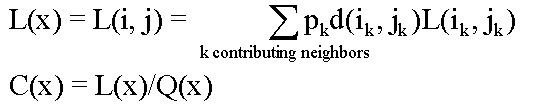Here d(x) = d(i, j) is a decay multiplier giving the fractional (first order) reduction in mass in moving from grid cell x to the next downslope cell. If travel (or residence) times t(x) associated with flow between cells are available d(x) may be evaluated as exp(-k t(x)) where k is a first order decay parameter. The Concentration grid output is C(x). If the outlets shapefile is used, the tool only evaluates the part of the domain that contributes flow to the locations given by the shapefile.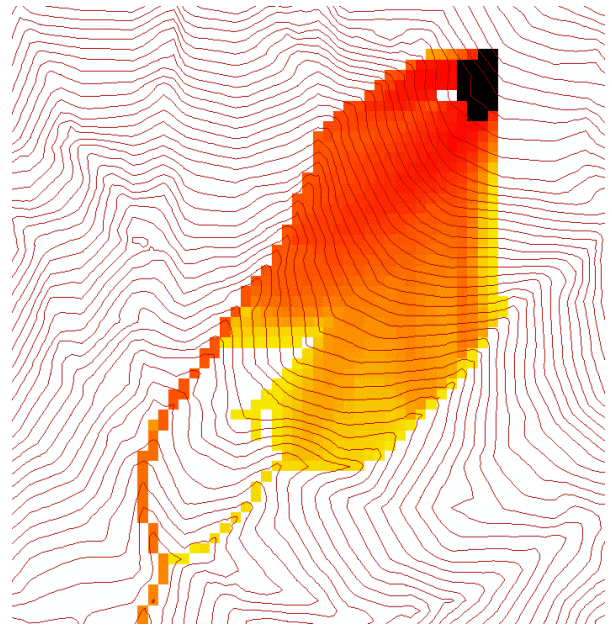Useful for a tracking a contaminant released or partitioned to flow at a fixed threshold concentration.

### Parameter¶

D-Infinity Fliessrichtung Raster [raster]
A grid giving flow direction by the D-infinity method. Flow direction is measured in radians, counter clockwise from east. This grid can be created by the function “D-Infinity Flow Directions”.
Disturbance Indicator Grid [raster]
A grid that indicates the source zone of the area of substance supply and must be 1 inside the zone and 0 or NODATA over the rest of the domain.
Decay Multiplier Grid [raster]
A grid giving the factor by which flow leaving each grid cell is multiplied before accumulation on downslope grid cells. This may be used to simulate the movement of an attenuating or decaying substance. If travel (or residence) times t(x) associated with flow between cells are available d(x) may be evaluated as exp(-k t(x)) where k is a first order decay parameter.
Effective Runoff Weight Grid [raster]
A grid giving the input quantity (notionally effective runoff or excess precipitation) to be used in the D-infinity weighted contributing area evaluation of Overland Flow Specific Discharge.
Auslaß-Shapedatei [vector: point]

Optional.

This optional input is a point shapefile defining outlets of interest. If this file is used, the tool will only evaluate the area upslope of these outlets.

Concentration Threshold [number]

The concentration or solubility threshold. Over the substance supply area, concentration is at this threshold.

Vorgabe: 1.0

Kantenverunreinigung prüfen [boolean]

This option determines whether the tool should check for edge contamination. Edge contamination is defined as the possibility that a value may be underestimated due to grid cells outside of the domain not being considered when determining contributing area.

Vorgabe: True

### Ausgaben¶

Concentration Grid [raster]
A grid giving the resulting concentration of the compound of interest in the flow.

### Console usage¶

```processing.runalg('taudem:dinfinityconcentrationlimitedaccumulation', -ang, -dg, -dm, -q, -o, -csol, -nc, -ctpt)
```

## D-Unendlich abnehmende Akkumulation¶

### Beschreibung¶

The D-Infinity Decaying Accumulation tool creates a grid of the accumulated quantity at each location in the domain where the quantity accumulates with the D-infinity flow field, but is subject to first order decay in moving from cell to cell. By default, the quantity contribution of each grid cell is the cell length to give a per unit width accumulation, but can optionally be expressed with a weight grid. The decay multiplier grid gives the fractional (first order) reduction in quantity in accumulating from grid cell x to the next downslope cell.

A decayed accumulation operator DA[.] takes as input a mass loading field m(x) expressed at each grid location as m(i, j) that is assumed to move with the flow field but is subject to first order decay in moving from cell to cell. The output is the accumulated mass at each location DA(x). The accumulation of m at each grid cell can be numerically evaluated.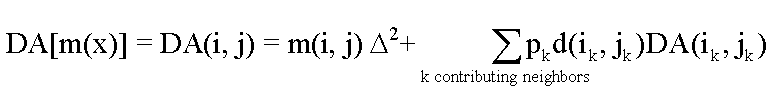Here d(x) = d(i ,j) is a decay multiplier giving the fractional (first order) reduction in mass in moving from grid cell x to the next downslope cell. If travel (or residence) times t(x) associated with flow between cells are available d(x) may be evaluated as exp(-k t(x)) where k is a first order decay parameter. The weight grid is used to represent the mass loading m(x). If not specified this is taken as 1. If the outlets shapefile is used the function is only evaluated on that part of the domain that contributes flow to the locations given by the shapefile.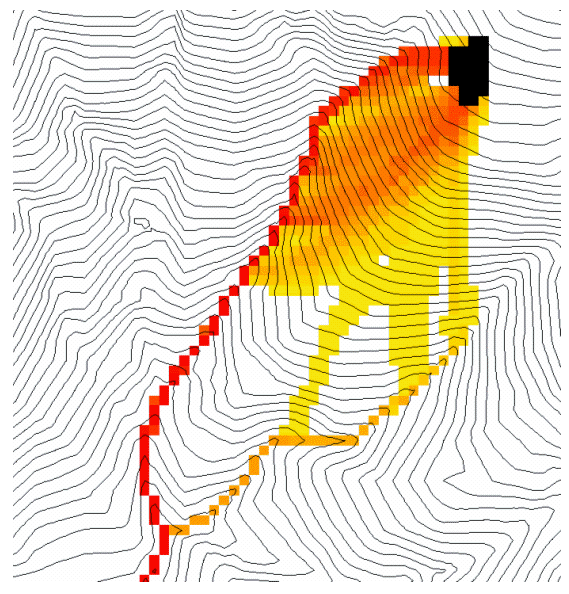Useful for a tracking contaminant or compound subject to decay or attenuation.

### Parameter¶

D-Infinity Fliessrichtung Raster [raster]
A grid giving flow direction by the D-infinity method. Flow direction is measured in radians, counter clockwise from east. This grid can be created by the function “D-Infinity Flow Directions”.
Decay Multiplier Grid [raster]
A grid giving the factor by which flow leaving each grid cell is multiplied before accumulation on downslope grid cells. This may be used to simulate the movement of an attenuating substance.
Weight Grid [raster]

Optional.

A grid giving weights (loadings) to be used in the accumulation. If this optional grid is not specified, weights are taken as the linear grid cell size to give a per unit width accumulation.

Auslaß-Shapedatei [vector: point]

Optional.

This optional input is a point shapefile defining outlets of interest. If this file is used, the tool will only evaluate the area upslope of these outlets.

Kantenverunreinigung prüfen [boolean]

This option determines whether the tool should check for edge contamination. Edge contamination is defined as the possibility that a value may be underestimated due to grid cells outside of the domain not being considered when determining contributing area.

Vorgabe: True

### Ausgaben¶

Decayed Specific Catchment Area Grid [raster]
The D-Infinity Decaying Accumulation tool creates a grid of the accumulated mass at each location in the domain where mass moves with the D-infinity flow field, but is subject to first order decay in moving from cell to cell.

### Console usage¶

```processing.runalg('taudem:dinfinitydecayingaccumulation', -ang, -dm, -wg, -o, -nc, -dsca)
```

## D-Infinity Entfernung nach unten¶

### Beschreibung¶

Calculates the distance downslope to a stream using the D-infinity flow model. The D-infinity flow model is a multiple flow direction model, because the outflow from each grid cell is proportioned between up to 2 downslope grid cells. As such, the distance from any grid cell to a stream is not uniquely defined. Flow that originates at a particular grid cell may enter the stream at a number of different cells. The statistical method may be selected as the longest, shortest or weighted average of the flow path distance to the stream. Also one of several ways of measuring distance may be selected: the total straight line path (Pythagoras), the horizontal component of the straight line path, the vertical component of the straight line path, or the total surface flow path.

### Parameter¶

D-Infinity Fliessrichtung Raster [raster]
A grid giving flow direction by the D-infinity method. Flow direction is measured in radians, counter clockwise from east. This can be created by the tool “D-Infinity Flow Directions”.
Höhengitter mit gefüllten Löchern [raster]
This input is a grid of elevation values. As a general rule, it is recommended that you use a grid of elevation values that have had the pits removed for this input. Pits are generally taken to be artifacts that interfere with the analysis of flow across them. This grid can be obtained as the output of the “Pit Remove” tool, in which case it contains elevation values where the pits have been filled to the point where they just drain.
Stromrastergitter [raster]
A grid indicating streams, by using a grid cell value of 1 on streams and 0 off streams. This is usually the output of one of the tools in the “Stream Network Analysis” toolset.

Optional.

A grid giving weights (loadings) to be used in the distance calculation. This might be used for example where only flow distance through a buffer is to be calculated. The weight is then 1 in the buffer and 0 outside it. Alternatively the weight may reflect some sort of cost function for travel over the surface, perhaps representing travel time or attenuation of a process. If this input file is not used, the loadings will assumed to be one for each grid cell.

Statistical Method [selection]

Statistical method used to calculate the distance down to the stream. In the D-Infinity flow model, the outflow from each grid cell is proportioned between two downslope grid cells. Therefore, the distance from any grid cell to a stream is not uniquely defined. Flow that originates at a particular grid cell may enter the stream at a number of cells. The distance to the stream may be defined as the longest (maximum), shortest (minimum) or weighted average of the distance down to the stream.

Optionen:

• 0 — Minimum
• 1 — Maximum
• 2 — Durchschnitt

Vorgabe: 2

Distance Method [selection]

Distance method used to calculate the distance down to the stream. One of several ways of measuring distance may be selected: the total straight line path (Pythagoras), the horizontal component of the straight line path (horizontal), the vertical component of the straight line path (vertical), or the total surface flow path (surface).

Optionen:

• 0 — Pythagoras
• 1 — Horizontal
• 2 — Vertikal

• 3 — Oberfläche

Vorgabe: 1

Kantenverunreinigung prüfen [boolean]

A flag that determines whether the tool should check for edge contamination. This is defined as the possibility that a value may be underestimated due to grid cells outside of the domain not being counted. In the context of Distance Down this occurs when part of a flow path traced downslope from a grid cell leaves the domain without reaching a stream grid cell. With edge contamination checking selected, the algorithm recognizes this and reports no data for the result. This is the desired effect and indicates that values for these grid cells is unknown due to it being dependent on terrain outside of the domain of data available. Edge contamination checking may be overridden in cases where you know this is not an issue or want to evaluate the distance using only the fraction of flow paths that terminate at a stream.

Vorgabe: True

### Ausgaben¶

D-Infinity Abstand nach unten [raster]
Grid containing the distance to stream calculated using the D-infinity flow model and the statistical and path methods chosen.

### Console usage¶

```processing.runalg('taudem:dinfinitydistancedown', dinf_flow_dir_grid, pit_filled_grid, stream_grid, weight_path_grid, stat_method, dist_method, edge_contam, dist_down_grid)
```

## D-Infinity Entfernung hoch¶

### Beschreibung¶

This tool calculates the distance from each grid cell up to the ridge cells along the reverse D-infinity flow directions. Ridge cells are defined to be grid cells that have no contribution from grid cells further upslope. Given the convergence of multiple flow paths at any grid cell, any given grid cell can have multiple upslope ridge cells. There are three statictical methods that this tool can use: maximum distance, minimum distance and waited flow average over these flow paths. A variant on the above is to consider only grid cells that contribute flow with a proportion greater than a user specified threshold (t) to be considered as upslope of any given grid cell. Setting t=0.5 would result in only one flow path from any grid cell and would give the result equivalent to a D8 flow model, rather than D-infinity flow model, where flow is proportioned between two downslope grid cells. Finally there are several different optional paths that can be measured: the total straight line path (Pythagoras), the horizontal component of the straight line path, the vertical component of the straight line path, or the total surface flow path.

### Parameter¶

D-Infinity Fliessrichtung Raster [raster]
A grid giving flow direction by the D-infinity method. Flow direction is measured in radians, counter clockwise from east. This can be created by the tool “D-Infinity Flow Directions”.
Höhengitter mit gefüllten Löchern [raster]
This input is a grid of elevation values. As a general rule, it is recommended that you use a grid of elevation values that have had the pits removed for this input. Pits are generally taken to be artifacts that interfere with the analysis of flow across them. This grid can be obtained as the output of the “Pit Remove” tool, in which case it contains elevation values where the pits have been filled to the point where they just drain.
Neigungsgitter [raster]
This input is a grid of slope values. This is measured as drop/distance and it is most often obtained as the output of the “D-Infinity Flow Directions” tool.
Statistical Method [selection]

Statistical method used to calculate the distance down to the stream. In the D-Infinity flow model, the outflow from each grid cell is proportioned between two downslope grid cells. Therefore, the distance from any grid cell to a stream is not uniquely defined. Flow that originates at a particular grid cell may enter the stream at a number of cells. The distance to the stream may be defined as the longest (maximum), shortest (minimum) or weighted average of the distance down to the stream.

Optionen:

• 0 — Minimum
• 1 — Maximum
• 2 — Durchschnitt

Vorgabe: 2

Distance Method [selection]

Distance method used to calculate the distance down to the stream. One of several ways of measuring distance may be selected: the total straight line path (Pythagoras), the horizontal component of the straight line path (horizontal), the vertical component of the straight line path (vertical), or the total surface flow path (surface).

Optionen:

• 0 — Pythagoras
• 1 — Horizontal
• 2 — Vertikal

• 3 — Oberfläche

Vorgabe: 1

Proportion Threshold [number]

The proportion threshold parameter where only grid cells that contribute flow with a proportion greater than this user specified threshold (t) is considered to be upslope of any given grid cell. Setting t=0.5 would result in only one flow path from any grid cell and would give the result equivalent to a D8 flow model, rather than D-Infinity flow model, where flow is proportioned between two downslope grid cells.

Vorgabe: 0.5

Kantenverunreinigung prüfen [boolean]

A flag that determines whether the tool should check for edge contamination. This is defined as the possibility that a value may be underestimated due to grid cells outside of the domain not being counted.

Vorgabe: True

### Ausgaben¶

D-Infinity Entfernung hoch [raster]
Grid containing the distances up to the ridge calculated using the D-Infinity flow model and the statistical and path methods chosen.

### Console usage¶

```processing.runalg('taudem:dinfinitydistanceup', dinf_flow_dir_grid, pit_filled_grid, slope_grid, stat_method, dist_method, threshold, edge_contam, dist_up_grid)
```

## D-Infinity umgekehrte Akkumulation¶

### Beschreibung¶

This works in a similar way to evaluation of weighted Contributing area, except that the accumulation is by propagating the weight loadings upslope along the reverse of the flow directions to accumulate the quantity of weight loading downslope from each grid cell. The function also reports the maximum value of the weight loading downslope from each grid cell in the Maximum Downslope grid.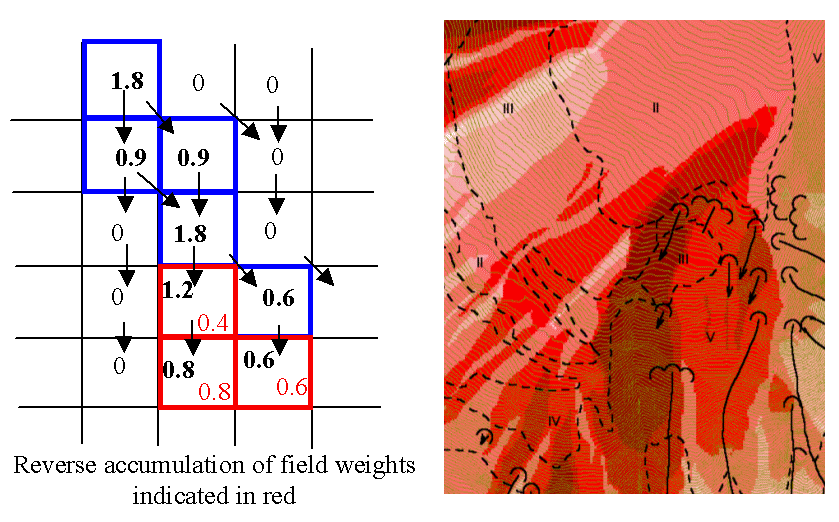This function is designed to evaluate and map the hazard due to activities that may have an effect downslope. The example is land management activities that increase runoff. Runoff is sometimes a trigger for landslides or debris flows, so the weight grid here could be taken as a terrain stability map. Then the reverse accumulation provides a measure of the amount of unstable terrain downslope from each grid cell, as an indicator of the danger of activities that may increase runoff, even though there may be no potential for any local impact.

### Parameter¶

D-Infinity Fliessrichtung Raster [raster]
A grid giving flow direction by the D-infinity method. Flow direction is measured in radians, counter clockwise from east. This can be created by the tool “D-Infinity Flow Directions”.
Weight Grid [raster]

### Ausgaben¶

Reverse Accumulation Grid [raster]
The grid giving the result of the “Reverse Accumulation” function. This works in a similar way to evaluation of weighted Contributing area, except that the accumulation is by propagating the weight loadings upslope along the reverse of the flow directions to accumulate the quantity of loading downslope from each grid cell.
Maximum Downslope Grid [raster]
The grid giving the maximum of the weight loading grid downslope from each grid cell.

### Console usage¶

```processing.runalg('taudem:dinfinityreverseaccumulation', -ang, -wg, -racc, -dmax)
```

## D-Infinity transportbegrenzte Akkumulation - 2¶

### Beschreibung¶

This function is designed to calculate the transport and deposition of a substance (e.g. sediment) that may be limited by both supply and the capacity of the flow field to transport it. This function accumulates substance flux (e.g. sediment transport) subject to the rule that transport out of any grid cell is the minimum between supply and transport capacity, Tcap. The total supply at a grid cell is calculated as the sum of the transport in from upslope grid cells, Tin, plus the local supply contribution, E (e.g. erosion). This function also outputs deposition, D, calculated as total supply minus actual transport.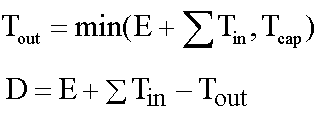Here E is the supply. Tout at each grid cell becomes Tin for downslope grid cells and is reported as Transport limited accumulation (tla). D is deposition (tdep). The function provides the option to evaluate concentration of a compound (contaminant) adhered to the transported substance. This is evaluated as follows: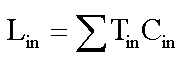Where Lin is the total incoming compound loading and Cin and Tin refer to the Concentration and Transport entering from each upslope grid cell.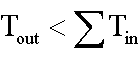If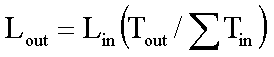else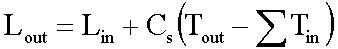where Cs is the concentration supplied locally and the difference in the second term on the right represents the additional supply from the local grid cell. Then,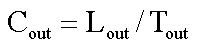Cout at each grid cell comprises is the concentration grid output from this function.

If the outlets shapefile is used the tool only evaluates that part of the domain that contributes flow to the locations given by the shapefile.

Transport limited accumulation is useful for modeling erosion and sediment delivery, including the spatial dependence of sediment delivery ratio and contaminant that adheres to sediment.

### Parameter¶

D-Infinity Fliessrichtung Raster [raster]
A grid giving flow direction by the D-infinity method. Flow direction is measured in radians, counter clockwise from east. This can be created by the tool “D-Infinity Flow Directions”.
Supply Grid [raster]
A grid giving the supply (loading) of material to a transport limited accumulation function. In the application to erosion, this grid would give the erosion detachment, or sediment supplied at each grid cell.
Transportkapazitätsgitter [raster]
A grid giving the transport capacity at each grid cell for the transport limited accumulation function. In the application to erosion this grid would give the transport capacity of the carrying flow.
Eingangs Konzentrationsgitter [raster]
A grid giving the concentration of a compound of interest in the supply to the transport limited accumulation function. In the application to erosion, this grid would give the concentration of say phosphorous adhered to the eroded sediment.
Auslaß-Shapedatei [vector: point]

Optional.

This optional input is a point shapefile defining outlets of interest. If this file is used, the tool will only evaluate the area upslope of these outlets.

Kantenverunreinigung prüfen [boolean]

This option determines whether the tool should check for edge contamination. Edge contamination is defined as the possibility that a value may be underestimated due to grid cells outside of the domain not being considered when determining the result.

Vorgabe: True

### Ausgaben¶

Transportbegrenztes Akkumulations Gitter [raster]
This grid is the weighted accumulation of supply accumulated respecting the limitations in transport capacity and reports the transport rate calculated by accumulating the substance flux subject to the rule that the transport out of any grid cell is the minimum of the total supply (local supply plus transport in) to that grid cell and the transport capacity.
Ablagerungs Raster [raster]
A grid giving the deposition resulting from the transport limited accumulation. This is the residual from the transport in to each grid cell minus the transport capacity out of the grid cell. The deposition grid is calculated as the transport in + the local supply - the tranport out.
Ausgangs Konzentrationsgitter [raster]
If an input concentation in supply grid is given, then this grid is also output and gives the concentration of a compound (contaminant) adhered or bound to the transported substance (e.g. sediment) is calculated.

### Console usage¶

```processing.runalg('taudem:dinfinitytransportlimitedaccumulation2', dinf_flow_dir_grid, supply_grid, capacity_grid, in_concentr_grid, outlets_shape, edge_contam, transp_lim_accum_grid, deposition_grid, out_concentr_grid)
```

## D-Infinity transportbegrenzte Akkumulation¶

### Beschreibung¶

This function is designed to calculate the transport and deposition of a substance (e.g. sediment) that may be limited by both supply and the capacity of the flow field to transport it. This function accumulates substance flux (e.g. sediment transport) subject to the rule that transport out of any grid cell is the minimum between supply and transport capacity, Tcap. The total supply at a grid cell is calculated as the sum of the transport in from upslope grid cells, Tin, plus the local supply contribution, E (e.g. erosion). This function also outputs deposition, D, calculated as total supply minus actual transport.Here E is the supply. Tout at each grid cell becomes Tin for downslope grid cells and is reported as Transport limited accumulation (tla). D is deposition (tdep). The function provides the option to evaluate concentration of a compound (contaminant) adhered to the transported substance. This is evaluated as follows:Where Lin is the total incoming compound loading and Cin and Tin refer to the Concentration and Transport entering from each upslope grid cell.Ifelsewhere Cs is the concentration supplied locally and the difference in the second term on the right represents the additional supply from the local grid cell. Then,Cout at each grid cell comprises is the concentration grid output from this function.

If the outlets shapefile is used the tool only evaluates that part of the domain that contributes flow to the locations given by the shapefile.

Transport limited accumulation is useful for modeling erosion and sediment delivery, including the spatial dependence of sediment delivery ratio and contaminant that adheres to sediment.

### Parameter¶

D-Infinity Fliessrichtung Raster [raster]
A grid giving flow direction by the D-infinity method. Flow direction is measured in radians, counter clockwise from east. This can be created by the tool “D-Infinity Flow Directions”.
Supply Grid [raster]
A grid giving the supply (loading) of material to a transport limited accumulation function. In the application to erosion, this grid would give the erosion detachment, or sediment supplied at each grid cell.
Transportkapazitätsgitter [raster]
A grid giving the transport capacity at each grid cell for the transport limited accumulation function. In the application to erosion this grid would give the transport capacity of the carrying flow.
Auslaß-Shapedatei [vector: point]

Optional.

This optional input is a point shapefile defining outlets of interest. If this file is used, the tool will only evaluate the area upslope of these outlets.

Kantenverunreinigung prüfen [boolean]

This option determines whether the tool should check for edge contamination. Edge contamination is defined as the possibility that a value may be underestimated due to grid cells outside of the domain not being considered when determining the result.

Vorgabe: True

### Ausgaben¶

Transportbegrenztes Akkumulations Gitter [raster]
This grid is the weighted accumulation of supply accumulated respecting the limitations in transport capacity and reports the transport rate calculated by accumulating the substance flux subject to the rule that the transport out of any grid cell is the minimum of the total supply (local supply plus transport in) to that grid cell and the transport capacity.
Ablagerungs Raster [raster]
A grid giving the deposition resulting from the transport limited accumulation. This is the residual from the transport in to each grid cell minus the transport capacity out of the grid cell. The deposition grid is calculated as the transport in + the local supply - the tranport out.

### Console usage¶

```processing.runalg('taudem:dinfinitytransportlimitedaccumulation', dinf_flow_dir_grid, supply_grid, capacity_grid, outlets_shape, edge_contam, transp_lim_accum_grid, deposition_grid)
```

## D-Unendlich Aufwärtsabhängigkeit¶

### Beschreibung¶

The D-Infinity Upslope Dependence tool quantifies the amount each grid cell in the domain contributes to a destination set of grid cells. D-Infinity flow directions proportion flow from each grid cell between multiple downslope grid cells. Following this flow field downslope the amount of flow originating at each grid cell that reaches the destination zone is defined. Upslope influence is evaluated using a downslope recursion, examining grid cells downslope from each grid cell, so that the map produced identifies the area upslope where flow through the destination zone originates, or the area it depends on, for its flow.

The figures below illustrate the amount each source point in the domain x (blue) contributes to the destination point or zone y (red). If the indicator weighted contributing area function is denoted I(y; x) giving the weighted contribution using a unit value (1) from specific grid cells y to grid cells x, then the upslope dependence is: D(x; y) = I(y; x).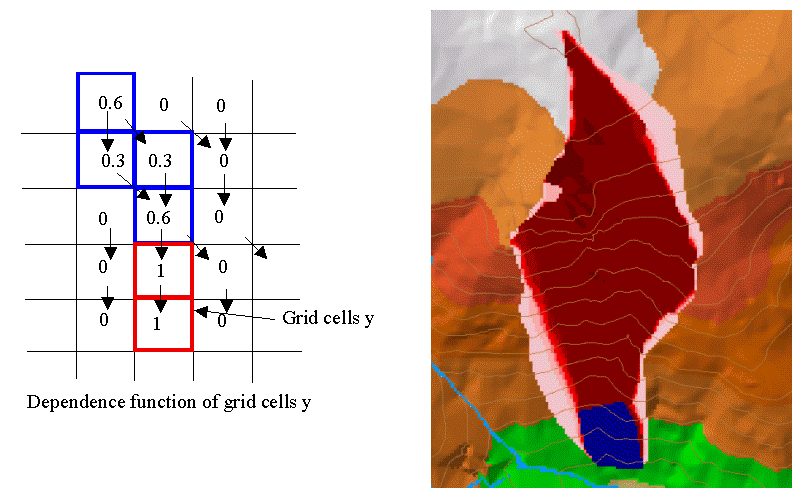This is useful for example to track where flow or a flow related substance or contaminant that enters a destination area may come from.

### Parameter¶

D-Infinity Fliessrichtung Raster [raster]
A grid giving flow direction by the D-Infinity method where the flow direction angle is determined as the direction of the steepest downward slope on the eight triangular facets formed in a 3x3 grid cell window centered on the grid cell of interest. This grid can be produced using the “D-Infinity Flow Direction” tool.
Zielgitter [raster]
A grid that encodes the destination zone that may receive flow from upslope. This grid must be 1 inside the zone y and 0 over the rest of the domain.

### Ausgaben¶

Output Upslope Dependence Grid [raster]
A grid quantifing the amount each source point in the domain contributes to the zone defined by the destination grid.

### Console usage¶

```processing.runalg('taudem:dinfinityupslopedependence', -ang, -dg, -dep)
```

## Gemitteltes Gefälle¶

### Beschreibung¶

This tool computes slope in a D8 downslope direction averaged over a user selected distance. Distance should be specified in horizontal map units.

### Parameter¶

D8 Fließrichtung Raster [raster]
This input is a grid of flow directions that are encoded using the D8 method where all flow from a cells goes to a single neighboring cell in the direction of steepest descent. This grid can be obtained as the output of the “D8 Flow Directions” tool.
Höhengitter mit gefüllten Löchern [raster]
This input is a grid of elevation values. As a general rule, it is recommended that you use a grid of elevation values that have had the pits removed for this input. Pits are generally taken to be artifacts that interfere with the analysis of flow across them. This grid can be obtained as the output of the “Pit Remove” tool, in which case it contains elevation values where the pits have been filled to the point where they just drain.
Downslope Distance [number]

Input parameter of downslope distance over which to calculate the slope (in horizontal map units).

Vorgabe: 50

### Ausgaben¶

Slope Average Down Grid [raster]
This output is a grid of slopes calculated in the D8 downslope direction, averaged over the selected distance.

### Console usage¶

```processing.runalg('taudem:slopeaveragedown', -p, -fel, -dn, -slpd)
```

## Gefälle-über-Flächenverhältnis¶

### Beschreibung¶

Calculates the ratio of the slope to the specific catchment area (contributing area). This is algebraically related to the more common ln(a/tan beta) wetness index, but contributing area is in the denominator to avoid divide by 0 errors when slope is 0.

### Parameter¶

Neigungsgitter [raster]
A grid of slope. This grid can be generated using ether the “D8 Flow Directions” tool or the “D-Infinity Flow Directions” tool.
Specific Catchment Area Grid [raster]
A grid giving the contributing area value for each cell taken as its own contribution plus the contribution from upslope neighbors that drain in to it. Contributing area is counted in terms of the number of grid cells (or summation of weights). This grid can be generated using either the “D8 Contributing Area” tool or the “D-Infinity Contributing Area” tool.

### Ausgaben¶

Slope Divided By Area Ratio Grid [raster]
A grid of the ratio of slope to specific catchment area (contributing area). This is algebraically related to the more common ln(a/tan beta) wetness index, but contributing area is in the denominator to avoid divide by 0 errors when slope is 0.

### Console usage¶

```processing.runalg('taudem:slopeoverarearatio', -slp, -sca, -sar)
```